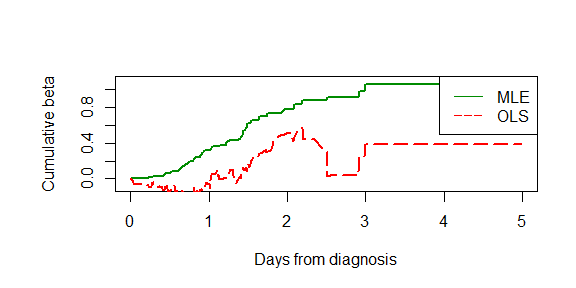# ahMLE_manual

### An R package for fitting the additive hazards model with maximum likelihood method.

#### What does the package do?

This R package implements several approaches to fitting the additive hazards model. Besides the traditional Aalen’s method, this package also offers the maximum likelihood methods to fit the model.

#### How does it work?

Given the survival variable (survival times and censors) and corresponding indpendent variables, the package offers the function ah which fits the coefficients of the independent variables as a step funtion of time by the choosing method.

For details, see: Maximum likelihood estimation in the additive hazards model(2021).

#### Attentions for the maximum likelihood method

By default, the package do not assume the range of the independent varaibles. However, for the maximum likelihood method, it will automatically take the maximum value and minimum value in the data as the range of the independent variable. If you do not want this setting – for example, you already know the ranges of the varialbes – please rearange the value of indpendent variables between $$[0,1]$$ by linear transformation and put the argument scale to be FALSE. You can calculate the true coefficients of the original data by the inverse of the former linear transformation manually.

When you use the maximum likelihood method with the default domain (the full domain which assures all hazard to be positive), we recommand you to use the optimial method given by the parameter ml_opt, which is also the default method. If you want to input your own domain matrix, the descending method given by the parameter ml_desc is recommanded. In this case, the comsuming time is very sensitive to the number of rows of the domain matrix. If you have very large number of domains (say more than $$100$$), it will comsume quite a lot of time.

## 1. Installing the package

ahMLE is available on CRAN and can be installed as follows in an R session:

install.packages("ahMLE")

Once installed, the package can be loaded in a given R session using:

library(ahMLE)

## 2. Running an analysis

We apply the method to the data from a clinical trial with 195 patients with carcinoma of the oropharynx by the Radiation Therapy Oncology Group in the United States. Patients were randomised into two treatment groups (“standard” and “experimental” treatment), and survival times were measured in days from diagnosis. Seven covariates were included in the data: Sex, Treatment, Grade, Age, Condition, T-stage and N-stage. The data is originally stored in the package invGauss(which is unforuntately out of update).

In our analysis, we take all the covariates as continuous. We re-scale them to $$[0,1]$$.


require(survival)
require(invGauss)
library(ahMLE)

data(d.oropha.rec)

SData = data.frame(time = d.oropha.rec$time, constant = 1, sex = d.oropha.rec$sex,
treatm = d.oropha.rec$treatm, grade = d.oropha.rec$grade,
age = d.oropha.rec$age, cond = d.oropha.rec$cond,
tstage = d.oropha.rec$tstage, nstage = d.oropha.rec$nstage,
event = d.oropha.rec$status) # Data rescaled SData$sex = SData$sex - 1 SData$treatm = SData$treatm -1 SData$grade = SData$grade -1 SData$age = (SData$age -60)/10 SData$cond = SData$cond -1 SData$tstage = SData$tstage - 1 With the data rescaled, we can fit the data with two methods, the Aalen’s OLS method and the maximum likelihood method. formula_input = Surv(time= time, event = event) ~ sex + treatm +grade + age +cond + tstage + nstage # Use Aalen's OLS method to compute the cumulative beta beta_aalen = ah(formula_input, data = SData, method = "aalen") Cbeta_aalen = beta_aalen$cumbeta

# Use (default) maximum likelihood method to compute the cumulative beta

beta_mle= ah(formula_input, data = SData, progbar = TRUE)
Cbeta_mle = beta_mle$cumbeta ## 3. Plot We illustrate the cumulative beta estimated by two methods for the additive hazards model.  old.par <- par(no.readonly = TRUE) par(mfrow=c(1,1)) par(mar=c(5, 6, 4, 2)) time_grid = beta_aalen$cumbeta[,1]
plot(time_grid,Cbeta_mle[,2],type="l", lwd = 2, col=colors(), ylim=c(-0.1,1.1),  ylab = "Cumulative beta",xlab = "Days from diagnosis", cex.lab=1)
lines(time_grid,Cbeta_aalen[,2], type="l", lty = 5, lwd =2, col="red")
legend("topright", legend=c("MLE","OLS"), col=c(colors(),"red"),lty=c(1,5), cex=1,lwd = 1)par(old.par)

## 4. Acknowledgement

The project is sponsored by the Leiden University Fund / Hakkenberg $$\alpha$$ $$\beta$$ $$\gamma$$-integratie Fonds, www.luf.nl. We would like to thank and acknowledge their support.

We acknowledge the authors of the package invGauss for importing their clinical data of carcinoma of the oropharynx.